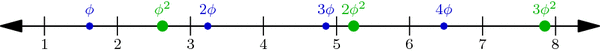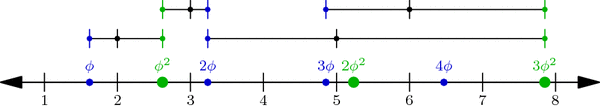# Just Beatty It

[This is the 6th post in the current series about Wythoff’s game: see posts #1, #2, #3, #4, and #5. Caveat lector: this post is a bit more difficult than usual. Let me know what you think in the comments!]

Our only remaining task from last week was to prove the mysterious Covering Theorem: we must show that there is exactly one dot in each row and column of the grid (we already covered the diagonal case). Since the rows and columns are symmetric, let’s focus on columns.

The columns really only care about the x-coordinates of the points, so let’s draw just these x-coordinates on the number-line. We’ve drawn $$\phi,2\phi,3\phi,\ldots$$ with small dots and $$\phi^2,2\phi^2,3\phi^2,\ldots$$ with large dots. We need to show that there’s exactly one dot between 1 and 2, precisely one dot between 2 and 3, just one between 3 and 4, and so on down the line. For terminology’s sake, break the number line into length-1 intervals [1,2], [2,3], [3,4], etc., so we must show that each interval has one and only one dot:Multiples of $$\phi$$ (small blue dots) and of $$\phi^2$$ (large green dots) perfectly interleave the integers on the number line. This is proved below.

Why is this true? One explanation hinges on a nice geometric observation: Take any small dot s and large dot t on our number-line above, and cut segment st into two parts in the ratio $$1:\phi$$ (with s on the shorter side). Then the point where we cut is always an integer! For example, the upper-left segment in the diagram below has endpoints at $$s=2\cdot\phi$$ and $$t=1\cdot\phi^2$$, and its cutting point is the integer 3:When a segment formed by a small and a large dot is cut into a $$1:\phi$$ ratio (closer to the small dot), the cutting point is always an integer. In fact, the segment between $$j\cdot \phi$$ and $$k\cdot \phi^2$$ is cut at the integer j+k.

In general, if s is the jth small dot—i.e., $$s=j\cdot\phi$$—and $$t=k\cdot\phi^2$$ is the kth large dot, then the cutting point between s and t is $$\frac{1}{\phi}\cdot s+\frac{1}{\phi^2}\cdot t = j+k$$ (Why?!). But more importantly, this observation shows that no interval has two or more dots: a small dot and a large dot can’t be in the same interval because they always have an integer between them!

So all we have to do now is prove that no interval is empty: for each integer n, some dot lies in the interval [n,n+1]. We will prove this by contradiction. What happens if no dot hits this interval? Then the sequence $$\phi,2\phi,3\phi,\ldots$$ jumps over the interval, i.e., for some j, the jth dot in the sequence is less than n but the (j+1)st is greater than n+1. Likewise, the sequence $$\phi^2,2\phi^2,3\phi^2,\ldots$$ jumps over the interval: its kth dot is less than n while its (k+1)st dot is greater than n+1:Illustrating the hypothetical situation where interval [n, n+1] contains no dot. This is used in a proof by contradiction to show that the interval in fact cannot be empty.

By our observation above on segment $$s=j\phi$$ and $$t=k\phi^2$$, we find that the integer j+k is less than n, so $$j+k\le n-1$$. Similarly, $$j+k+2 > n+1$$, so $$j+k+2 \ge n+2$$. But together these inequalities say that $$n\le j+k\le n-1$$, which is clearly absurd! This is the contradiction we were hoping for, so the interval [n,n+1] is in fact not empty. This completes our proof of the Covering Theorem and the Wythoff formula!

It was a long journey, but we’ve finally seen exactly why the Wythoff losing positions are arranged as they are. Thank you for following me through this!

### A Few Words on the Column Covering Theorem

Using the floor function $$\lfloor x\rfloor$$ that rounds x down to the nearest integer, we can restate the Column Covering Theorem in perhaps a more natural context. The sequence of integers $$\lfloor\phi\rfloor = 1, \lfloor 2\phi\rfloor = 3, \lfloor 3\phi\rfloor = 4, \lfloor 4\phi\rfloor = 6, \ldots$$ is called the Beatty sequence for the number $$\phi$$, and similarly, $$\lfloor\phi^2\rfloor = 2, \lfloor 2\phi^2\rfloor = 5, \lfloor 3\phi^2\rfloor = 7, \lfloor 4\phi^2\rfloor = 8,\ldots$$ is the Beatty sequence for $$\phi^2$$. Today we proved that these two sequence are complementary, i.e., together they contain each positive integer exactly once. We seemed to use very specific properties of the numbers $$\phi$$ and $$\phi^2$$, but in fact, a much more general theorem is true:

Beatty’s Theorem: If $$\alpha$$ and $$\beta$$ are any positive irrational numbers with $$\frac{1}{\alpha}+\frac{1}{\beta}=1$$, then their Beatty sequences $$\lfloor\alpha\rfloor, \lfloor 2\alpha\rfloor, \lfloor 3\alpha\rfloor,\ldots$$ and $$\lfloor\beta\rfloor, \lfloor 2\beta\rfloor, \lfloor 3\beta\rfloor,\ldots$$ are complementary sequences.

Furthermore, our same argument—using $$\alpha$$ and $$\beta$$ instead of $$\phi$$ and $$\phi^2$$—can be used to prove the more general Beatty’s Theorem!

### Notes

1. Hint: use the identity $$\frac{1}{\phi}+\frac{1}{\phi^2}=1$$. []
2. To be thorough, we should also check that no interval has two small or two large dots. Why can’t this happen? []